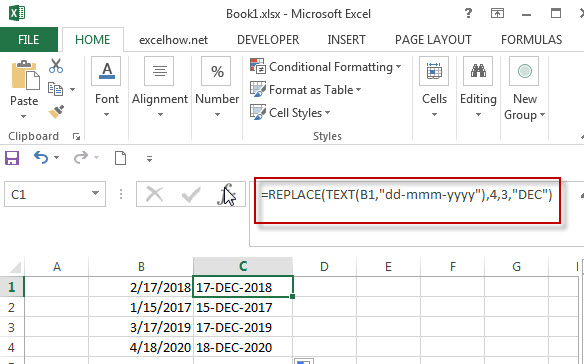# Combining the Replace function with Text function in Excel

In excel, we can use Replace function in combination with Text function to replace date characters that are part of the text string. And this post will guide you how to use TEXT function as the first arguments to replace text string in REPLACE function in excel 2013.

We have talked in the previous post that the TEXT function will return a text string, so the returned result of Text function can be as the first argument in the REPLACE function.

The Excel REPLACE function replaces all or part of a text string with another text string

The syntax of the REPLACE function is as below:

`= REPLACE(old_text, start_num, num_chars, new_text)`

## Combining the Replace function with Text function

Assuming that there is a date list in Column B, and you want to replace all month name to “DEC”.  As the date is recognized as numeric value in excel, you must convert the date as text string using TEXT function.  So we can write down the following REPLACE function:

`=REPLACE(TEXT(B1,”dd-mmm-yyyy”),4,3,”DEC”)`

We can enter the above REPLACE formula into Cell B1, then drag the Fill Handle to the entire Column B. it will apply for the other cells for this formula. Then you will get the expected results. Let’s see the following screenshot:### Related Functions

• Excel Replace function
The Excel REPLACE function replaces all or part of a text string with another text string.The syntax of the REPLACE function is as below:=REPLACE (old_text, start_num, num_chars, new_text)…
• Excel Text function
The Excel TEXT function converts a numeric value into text string with a specified format. The TEXT function is a build-in function in Microsoft Excel and it is categorized as a Text Function. The syntax of the TEXT function is as below: = TEXT (value, Format code)…
Related Posts

To pad a number with zeros, you need to add leading spaces and/or carriers. For example, if the original digit contains 2 digits, three additional characters will be added on before it. If there are 3 digits in total, allocating ...

How to Auto Fill Weekdays or Weekends in Excel

Sometimes we may want to enter a sequence of days in excel for some purpose, and we can press Ctrl+; to insert current date into cell, and then drag the cell down to attach following days into other cells, then ...

How to Change Date to The Day of Week in Excel

In excel, we can type a date properly if set cell format as ‘Date’. If there is a list includes multiple dates, is there any way to convert these dates to the days of week accordingly? Actually, there are some ...

How to Create Increment Number with Texts in Excel

Sometimes we want to fill 1,2,3,4,5… into each cell in a column, for implement this we can enter 1 in the first cell and then drag fill handle down to fill the following cells. But if there are some texts ...

How to Generate Random Time in Excel

In some special situations we may need to generate some random times in worksheet. We can enter time manually and one by one, but if we want to require a lot of random dates in excel worksheet, we will spend ...

How to Combine Text and Date into one Cell in Excel

This post will guide you how to combine text and dates in the same cell in Excel. How do I combine text and date values into one Cell in Excel 2013/2016. Combine Text and Date into One Cell Assuming that ...

How to Highlight Cell or Row If Date Is In Current Day/Week/Month in Excel

This post will guide you how to highlight cell if date is the current day or is in the current week or month in Excel. How do I highlight row if date is in current week or month with conditional ...

How to Convert Military Time to Standard Time in Excel

This post will guide you how to convert military time to standard time with a formula in Excel. How do I convert from military time 17:29:30 to regular time 5:29:30PM in Excel.  How do I Convert Standard time to military ...

How to Delete or Remove Year from a Date in Excel

This post will guide you how to delete or remove year from a given standard date in Excel. How do I remove the year from a date with a formula in Excel. Remove Year from a Date Assuming that you ...

How to Convert Date to YYYY-MM-DD format in Excel

This post will guide you how to convert the current date to a specified date format in Excel. How do I convert date to YYYY-MM-DD format with Format Cells Feature in Excel. How to convert date format to a specific ...

Sidebar